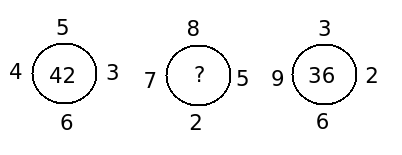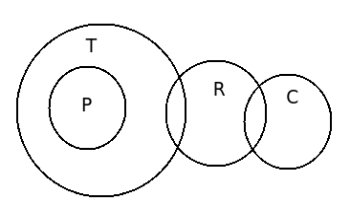# Model Aptitude questions and answers for placement - Set 17

1)   Arrange the following in a meaningful sequence :

1. Letter
2. Sentence
3. Word
4. Phrase

a. 1, 2, 4, 3
b. 2, 1, 3, 4
c. 2, 4, 1, 3
d. 1, 3, 4, 2
e. None of these
 Answer  Explanation ANSWER: 1, 3, 4, 2 Explanation: A group of letters makes a word. A group of words makes a phrase. A group of phrases makes a sentence.Thus, correct order is 1, 3, 4, 2.

2)   Three numbers are in the ratio 4 : 5 : 6 and their average is 30 . The largest number is:

a. 28
b. 32
c. 36
d. 42
 Answer  Explanation ANSWER: 36 Explanation: Let the numbers be 4x, 5x and 6x. Therefore, (4x+5x+6x)/3 = 3015x = 90x = 6Largest number = 6x = 36.

3)   At present, the ratio between the ages of Amit and Dhiraj is 5 : 4. After 6 years, Amit’s age will be 26 years. What is the age of Dhiraj at present?

a. 12 years
b. 16 years
c. 19 ½ years
d. 21 years
 Answer  Explanation ANSWER: 16 years Explanation: Let the present ages of Amit and Dhiraj be 5x years and 4x years respectively. Then,5x + 6 = 265x = 20x = 4Dhiraj’s age = 4x = 16 years

4)   Find the missing numbera. 50
b. 51
c. 48
d. 53
e. 55
 Answer  Explanation ANSWER: 51 Explanation: We have :(5 * 6) + (4 * 3) = 30 + 12 = 42(3 * 6) + (9 * 2) = 18 + 18 = 36Therefore, the missing number is (8 * 2) + (7 * 5) = 16 + 35 = 51

5)   Find the value of X.
(15)3.5 * (15)x = 158

a. 2.29
b. 2.75
c. 4.25
d. 4.5
 Answer  Explanation ANSWER: 4.5 Explanation: Let (15)3.5 * (15)x = 158Then, (15)3.5 + x = (15)83.5 + x = 8x = (8 – 3.5)x = 4.5

6)   Arrange the given words in the sequence in which they occur in the dictionary and choose the correct sequence.

1. Economic
2. Earlier
3. Each
4. Edition
5. Eager

a. 3, 4, 1, 5, 2
b. 2, 1, 3, 5, 4
c. 3, 2, 4, 1, 5
d. 3, 5, 2, 1, 4
e. None of these
 Answer  Explanation ANSWER: 3, 5, 2, 1, 4 Explanation: The correct alphabetical order is Each, Eager, Earlier, Economic, Edition

7)   If the price of rice rises from Rs. 6 per kg to Rs. 8 per kg, to have no increase in his expenditure on rice, a person will have to reduce his consumption of sugar by

a. 12 %
b. 19 %
c. 25 %
d. 32 %
 Answer  Explanation ANSWER: 25 % Explanation: Let original consumption = 100 kg and consumption = y kg.So, 100 x 6 = y x 8y = 75 kgReduction in consumption = 25 %.

8)   Two trains of lengths 156.62 and 100 meters are running on parallel lines with respective speeds of 30 km/hr and 36 km/hr. The time of crossing each other, if they run in the opposite direction is __________ .

a. 10 sec
b. 62 sec
c. 14 sec
d. 12 sec
e. None of these
 Answer  Explanation ANSWER: 14 sec Explanation: Total distance to be covered = 156.62 + 100 = 256.62 mTrains are running in opposite directions, henceRelative speed = 30 + 36 = 66 km/hr = 18.33 m/sec Time = Distance / Relative Speed = 256.62 /18.33 sec= 14 secTherefore, the time of crossing each other in the opposite direction is 14 seconds.

9)   Choose the number pair/group which is different from others.

1. 40 : 50
2. 30 : 36
3. 8 : 10
4. 28 : 35
5. 16 : 20

a. 8 : 10
b. 40 : 50
c. 30 : 36
d. 28 : 35
e. 16 : 20
 Answer  Explanation ANSWER: 30 : 36 Explanation: 40 : 50 ==> 4 : 530 : 36 ==> 5 : 68 : 10 ==> 4 : 528 : 35 ==> 4 : 516 : 20 ==> 4 : 5In each of the pair except 30 : 36, the ratio of the two numbers is 4 : 5.

10)   A man buys 50 pens at marked price of 46 pens from a whole seller. If he sells these pens giving a discount of 1% , what is the profit percent?

a. 5.3 %
b. 7.6 %
c. 10.6 %
d. 12 %
 Answer  Explanation ANSWER: 7.6 % Explanation: Let Marked price be Re. 1 eachC.P. of 50 pens = Rs. 46 S.P. of 50 pens = 99% of Rs. 50 = Rs. 49.50Profit % = (Profit /C.P.) x 100Profit% = (3.50/46) x 100 = 7.6 %

11)   A textile worker’s wages are increased by 10% and afterwards decreased by 10 %. Find the change percentage in his wages.

a. 2 % increase
b. 4% increase
c. 1% decrease
d. 4% decrease
e. None of these
 Answer  Explanation ANSWER: 1% decrease Explanation: Let workers wages = XA 10% increase would mean new wages= (110/100) XA 10% decrease would mean new wages= (90/100) (110/100 X)= 99/100 X which means a decrease of 1 %

12)   Assume that there are three statements followed by several conclusions. You have to take the given statements to be true, even if they seem to be at variance from commonly-known facts and then decide which of the given conclusion/s logically follows.

Statements :  I. All Pens are Tables
II. Some tables are rulers.
III. Some rulers are chalk.

Conclusion :  I. Some tables are chalk.
II. Some pens are chalk.
III. Some rulers are pens.
IV. Some chalk are rulers

a. Only IV follows
b. Only II and I follows.
c. Only III follows.
d. Only I, II and III follows
e. None of these
 Answer  Explanation ANSWER: Only IV follows Explanation:This diagram tells us that only one condition follows i.e. some chalk are rulers.